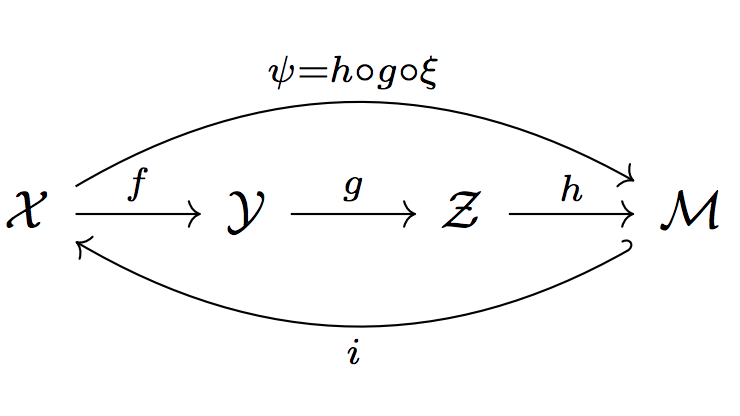# Topological Constraints on Homeomorphic Auto-Encoding

NeurIPS 2018, Workshop on Integration of Deep Learning Theories# Topological Constraints on Homeomorphic Auto-Encoding

NeurIPS 2018, Workshop on Integration of Deep Learning Theories### Abstract

When doing representation learning on data that lives on a known non-trivial manifold embedded in high dimensional space, it is natural to desire the encoder to be homeomorphic when restricted to the manifold, so that it is bijective and continuous with a continuous inverse. Using topological arguments, we show that when the manifold is non-trivial, the encoder must be globally discontinuous and propose a universal, albeit impractical, construction. In addition, we derive necessary constraints which need to be satisfied when designing manifold-specific practical encoders. These are used to analyse candidates for a homeomorphic encoder for the manifold of 3D rotations SO(3).

### Abstract

When doing representation learning on data that lives on a known non-trivial manifold embedded in high dimensional space, it is natural to desire the encoder to be homeomorphic when restricted to the manifold, so that it is bijective and continuous with a continuous inverse. Using topological arguments, we show that when the manifold is non-trivial, the encoder must be globally discontinuous and propose a universal, albeit impractical, construction. In addition, we derive necessary constraints which need to be satisfied when designing manifold-specific practical encoders. These are used to analyse candidates for a homeomorphic encoder for the manifold of 3D rotations SO(3).